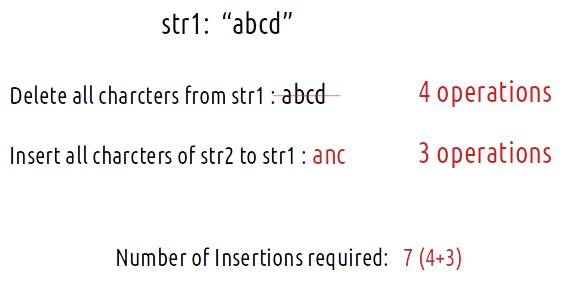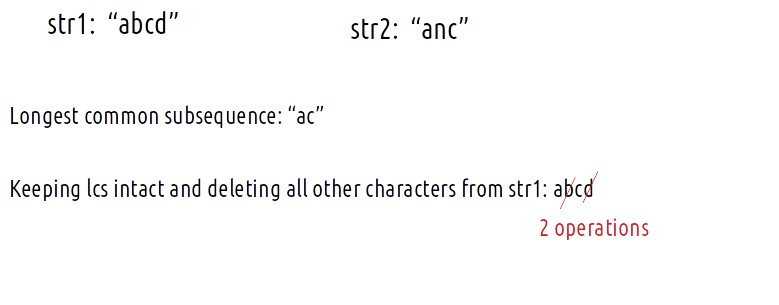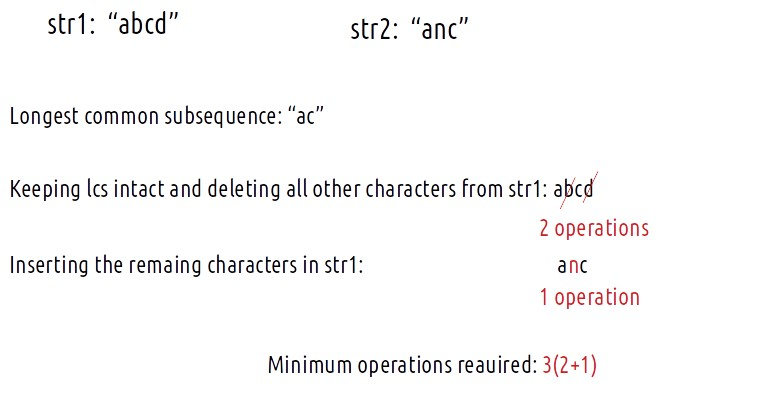# Minimum Insertions/Deletions to Convert String | (DP- 30)

Problem Statement: Minimum Insertions/Deletions to Convert String A to String B

We are given two strings, str1 and str2. We are allowed the following operations:

• Delete any number of characters from string str1.
• Insert any number of characters in string str1.

We need to tell the minimum operations required to convert str1 to str2.

```Example:```

Problem Link: Can You Make

### Solution :

Pre-req:  Longest Common Subsequence

### Intuition:

We need to find the minimum operations required to convert string str1 to str2. Let us keep the “minimum” criteria aside and think, what maximum operations will be required for this conversion?

The easiest way is to remove all the characters of str1 and then insert all the characters of str2. In this way, we will convert str1 to str2 with ‘n+m’ operations. (Here n and m are the length of strings str1 and str2 respectively).The problem states us to find the minimum of insertions. Let us try to figure it out:

• To minimize the operations, we will first try to refrain from deleting those characters which are already present in str2. More extensively, we refrain from deleting those characters which are common and come in the same order. To minimize the operations, we would like to keep the maximum common characters coming in the same order intact. These maximum characters are the characters of the longest common subsequence
• We will first keep the longest common subsequence of the str1 and str2 intact in str1 and delete all other characters from str1.• Next, we will insert all the remaining characters of str2 to str1.In order to minimize the operations, we need to find the length of the longest common subsequence.

Minimum Operations required = (n – k) + (m – k)

Here n and m are the length of str1 and str2 respectively and k is the length of the longest common subsequence of str1 and str2.

Approach:

The algorithm is stated as follows:

• Let n and m be the length of str1 and str2 respectively.
• Find the length of the longest common subsequence ( say k) of str1 and str2 as discussed in  Longest Common Subsequence.
• Return (n-k) + (m-k) as answer.

Code:

## C++ Code

``````#include <bits/stdc++.h>

using namespace std;

int lcs(string s1, string s2) {

int n=s1.size();
int m=s2.size();

vector<vector<int>> dp(n+1,vector<int>(m+1,-1));
for(int i=0;i<=n;i++){
dp[i] = 0;
}
for(int i=0;i<=m;i++){
dp[i] = 0;
}

for(int ind1=1;ind1<=n;ind1++){
for(int ind2=1;ind2<=m;ind2++){
if(s1[ind1-1]==s2[ind2-1])
dp[ind1][ind2] = 1 + dp[ind1-1][ind2-1];
else
dp[ind1][ind2] = 0 + max(dp[ind1-1][ind2],dp[ind1][ind2-1]);
}
}

return dp[n][m];
}

int canYouMake(string str1, string str2){

int n = str1.size();
int m = str2.size();

int k = lcs(str1,str2);

return (n-k)+(m-k);
}

int main() {

string str1= "abcd";
string str2= "anc";

cout<<"The Minimum operations required to convert str1 to str2: "<<
canYouMake(str1,str2);
}
``````

Output:

The Minimum operations required to convert str1 to str2: 3

Time Complexity: O(N*M)

Reason: There are two nested loops

Space Complexity: O(N*M)

Reason: We are using an external array of size (N*M). Stack Space is eliminated.

## Java Code

``````import java.util.*;

class TUF{
static int lcs(String s1, String s2) {

int n=s1.length();
int m=s2.length();

int dp[][]=new int[n+1][m+1];
for(int rows[]: dp)
Arrays.fill(rows,-1);
for(int i=0;i<=n;i++){
dp[i] = 0;
}
for(int i=0;i<=m;i++){
dp[i] = 0;
}

for(int ind1=1;ind1<=n;ind1++){
for(int ind2=1;ind2<=m;ind2++){
if(s1.charAt(ind1-1)==s2.charAt(ind2-1))
dp[ind1][ind2] = 1 + dp[ind1-1][ind2-1];
else
dp[ind1][ind2] = 0 + Math.max(dp[ind1-1][ind2],dp[ind1][ind2-1]);
}
}

return dp[n][m];
}

static int canYouMake(String str1, String str2){

int n = str1.length();
int m = str2.length();

int k = lcs(str1,str2);

return (n-k)+(m-k);
}

public static void main(String args[]) {

String str1= "abcd";
String str2= "anc";
System.out.println("The Minimum operations required to convert str1 to str2: "
+canYouMake(str1,str2));
}
}``````

Output:

The Minimum operations required to convert str1 to str2: 3

Time Complexity: O(N*M)

Reason: There are two nested loops

Space Complexity: O(N*M)

Reason: We are using an external array of size (N*M). Stack Space is eliminated.

Space Optimization

If we closely we are using two rows: dp[ind1-1][ ], dp[ind][ ],

So we are not required to contain an entire array, we can simply have two rows prev and cur where prev corresponds to dp[ind-1] and cur to dp[ind].

After declaring prev and cur, replace dp[ind-1] to prev and dp[ind] with cur and after the inner loop executes, we will set prev = cur, so that the cur row can serve as prev for the next index.

Code:

## C++ Code

``````#include <bits/stdc++.h>

using namespace std;

int lcs(string s1, string s2) {

int n=s1.size();
int m=s2.size();

vector<int> prev(m+1,0), cur(m+1,0);

// Base Case is covered as we have initialized the prev and cur to 0.

for(int ind1=1;ind1<=n;ind1++){
for(int ind2=1;ind2<=m;ind2++){
if(s1[ind1-1]==s2[ind2-1])
cur[ind2] = 1 + prev[ind2-1];
else
cur[ind2] = 0 + max(prev[ind2],cur[ind2-1]);
}
prev= cur;
}

return prev[m];
}

int canYouMake(string str1, string str2){

int n = str1.size();
int m = str2.size();

int k = lcs(str1,str2);

return (n-k)+(m-k);
}

int main() {

string str1= "abcd";
string str2= "anc";

cout<<"The Minimum operations required to convert str1 to str2: "<<
canYouMake(str1,str2);
}
``````

Output:

The Minimum operations required to convert str1 to str2: 3

Time Complexity: O(N*M)

Reason: There are two nested loops.

Space Complexity: O(M)

Reason: We are using an external array of size ‘M+1’ to store only two rows.

## Java Code

``````import java.util.*;

class TUF{
static int lcs(String s1, String s2) {

int n=s1.length();
int m=s2.length();

int[] prev=new int[m+1];
int[] cur=new int[m+1];

// Base Case is covered as we have initialized the prev and cur to 0.

for(int ind1=1;ind1<=n;ind1++){
for(int ind2=1;ind2<=m;ind2++){
if(s1.charAt(ind1-1)==s2.charAt(ind2-1))
cur[ind2] = 1 + prev[ind2-1];
else
cur[ind2] = 0 + Math.max(prev[ind2],cur[ind2-1]);
}
prev= cur;
}

return prev[m];
}

static int canYouMake(String str1, String str2){

int n = str1.length();
int m = str2.length();

int k = lcs(str1,str2);

return (n-k)+(m-k);
}

public static void main(String args[]) {

String str1= "abcd";
String str2= "anc";
System.out.println("The Minimum operations required to convert str1 to str2: "+
canYouMake(str1,str2));
}
}``````

Output:

The Minimum operations required to convert str1 to str2: 3

Time Complexity: O(N*M)

Reason: There are two nested loops.

Space Complexity: O(M)

Reason: We are using an external array of size ‘M+1’ to store only two rows.

Special thanks to Anshuman Sharma for contributing to this article on takeUforward. If you also wish to share your knowledge with the takeUforward fam, please check out this article